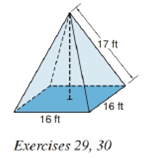Chapter 9.2, Problem 29EElementary Geometry For College St...

7th Edition
Alexander + 2 others
ISBN: 9781337614085

Solutions

Chapter
SectionElementary Geometry For College St...

7th Edition
Alexander + 2 others
ISBN: 9781337614085
Textbook Problem

A church steeple in the shape of a regular square pyramid needs to be reshingled. The part to be covered corresponds to the lateral area of the square pyramid. If each lateral edge measures 17 f t and each base edge measures 16 f t , how many square feet of shingles need to be replaced?To determine

To find:

The lateral area of the pyramid.

Explanation

Given:

A regular square pyramid as below,

and lateral edge measures 17ft and each base edge measures 16ft.

Properties Used:

A pyramid is made by connecting a base to an apex. The base is flat with straight edges, no curves, hence, a polygon and all other faces are triangles.

A regular pyramid is a pyramid whose base is a regular polygon and whose lateral edges are all congruent.

The lateral area L of a regular pyramid with slant height of length l and perimeter P of the base is given by

L=12lP.

The total surface area T of a pyramid with lateral area L and base area B is given by

T=L+B.

According to the Pythagorean theorem, in a right-angled triangle

hypotenuse2=base2+perpendicular2.

In a regular pyramid, the lengths of the apothem a of the base, the altitude h, and the slant height l satisfy the Pythagorean Theorem; that is, l2=a2+h2.

The perimeter of the square is four times the side.

Calculation:

From the given figure

Still sussing out bartleby?

Check out a sample textbook solution.

See a sample solution

The Solution to Your Study Problems

Bartleby provides explanations to thousands of textbook problems written by our experts, many with advanced degrees!

Get Started

Find more solutions based on key concepts